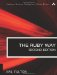# Section 5.27. Finding a Correlation Coefficient

### 5.26. Variance and Standard Deviation

The variance of a set of data is a measure of how "spread out" the values are. (Here we do not distinguish between biased and unbiased estimates.) The standard deviation, usually represented by a sigma (σ) is simply the square root of the variance.

`data = [2, 3, 2, 2, 3, 4, 5, 5, 4, 3, 4, 1, 2] def variance(x)   m = mean(x)   sum = 0.0   x.each {|v| sum += (v-m)**2 }   sum/x.size end def sigma(x)   Math.sqrt(variance(x)) end puts variance(data)   # 1.461538462 puts sigma(data)      # 1.20894105`

Note that the variance function in the preceding code uses the mean function defined earlier.The Ruby Way, Second Edition: Solutions and Techniques in Ruby Programming (2nd Edition)
ISBN: 0672328844
EAN: 2147483647
Year: 2004
Pages: 269
Authors: Hal Fulton

Similar book on Amazon